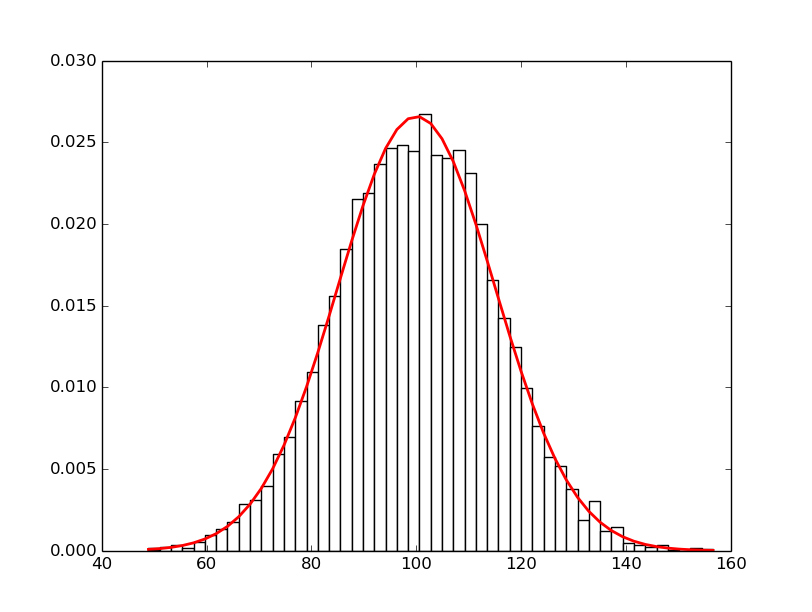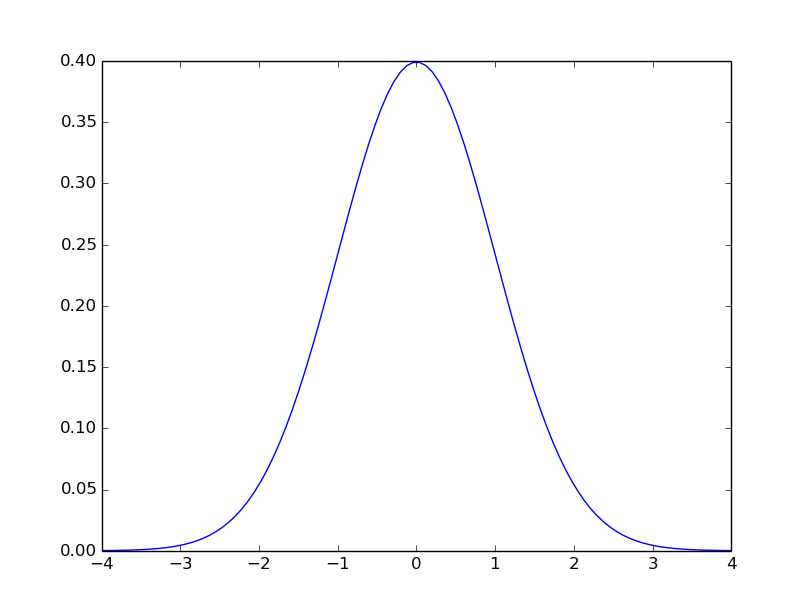## Normal distribution

Date: February 27th 2016
Last updated: February 27th 2016

You can produce a normal distribution using mean and standard deviation, or by using mean zero and sigma.

Mean and SD

``````import matplotlib.pyplot as plt
import matplotlib.mlab as mlab
from random import gauss

mu, sigma = 100, 15
x = [gauss(mu, sigma) for i in range(10000)]

# histogram
n, bins, patches = plt.hist(x, 50, normed=1, facecolor='white')

# pdf
pdf = mlab.normpdf(bins, mu, sigma)
plt.plot(bins, pdf, 'r-', linewidth=2)

plt.show()
``````Mean zero and sigma

``````import matplotlib.pyplot as plt
import matplotlib.mlab as mlab
import numpy as np

mu, sigma = 0, 1.0
x = np.linspace(-4, 4, 100)
plt.plot(x, mlab.normpdf(x, mu, sigma))
plt.show()
``````